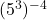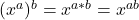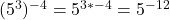## In which expression should the exponents be multiplied? (53)−4 one third to the 4th times one third to the 7th 3 to the 7th over 3 to the 15

Question

In which expression should the exponents be multiplied? (53)−4 one third to the 4th times one third to the 7th 3 to the 7th over 3 to the 15th 45 ⋅ 42

in progress 0
3 months 2021-08-12T23:21:39+00:00 2 Answers 46 views 0.

Step-by-step explanation:

According to the power rule, exponents can be multiplied when we have a number base that has an exponents which is raised to another power.

The following illustrates how to apply the power rule where multiplication of exponents is to be done:, where, x is the base number, a and b are exponents respectively.

From the options given, the expression where the exponents are required to be multiplied is.